#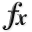N-D Test Functions L¶

class go_benchmark.Langermann(dimensions=2)

Langermann test objective function.

This class defines the Langermann global optimization problem. This is a multimodal minimization problem defined as follows: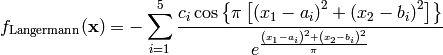Here,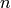represents the number of dimensions and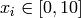for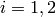.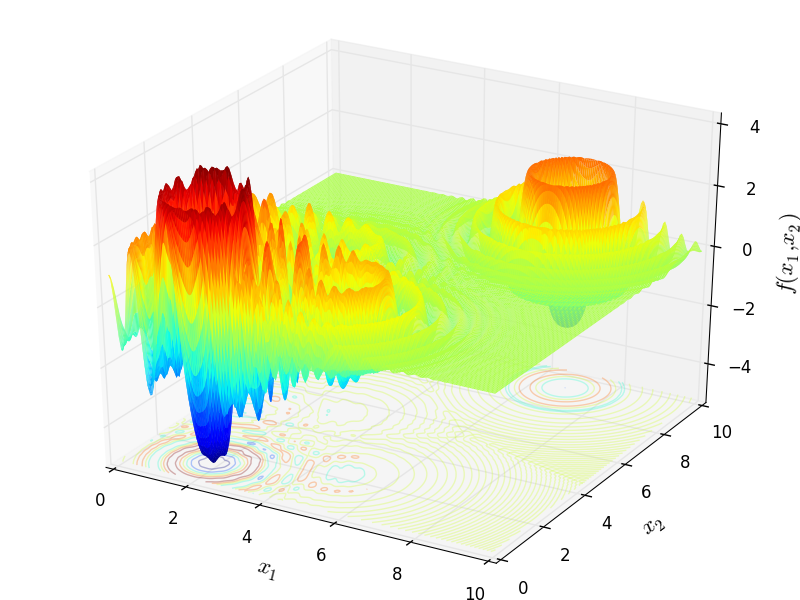Two-dimensional Langermann function

Global optimum: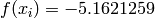for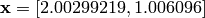class go_benchmark.LennardJones(dimensions=6)

LennardJones test objective function.

This class defines the Lennard-Jones global optimization problem. This is a multimodal minimization problem defined as follows: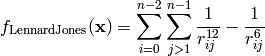Where, in this exercise: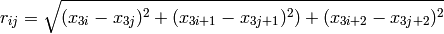Valid for any dimension,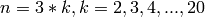.is the number of atoms in 3-D space constraints: unconstrained type: multi-modal with one global minimum; non-separable

Value-to-reach:. See array of minima below; additional minima available at the Cambridge cluster database:

http://www-wales.ch.cam.ac.uk/~jon/structures/LJ/tables.150.html

Here,represents the number of dimensions and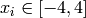for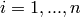.

Global optimum: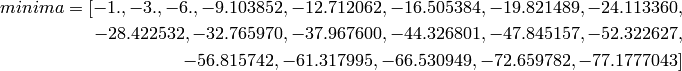class go_benchmark.Leon(dimensions=2)

Leon test objective function.

This class defines the Leon global optimization problem. This is a multimodal minimization problem defined as follows:Here,represents the number of dimensions andfor.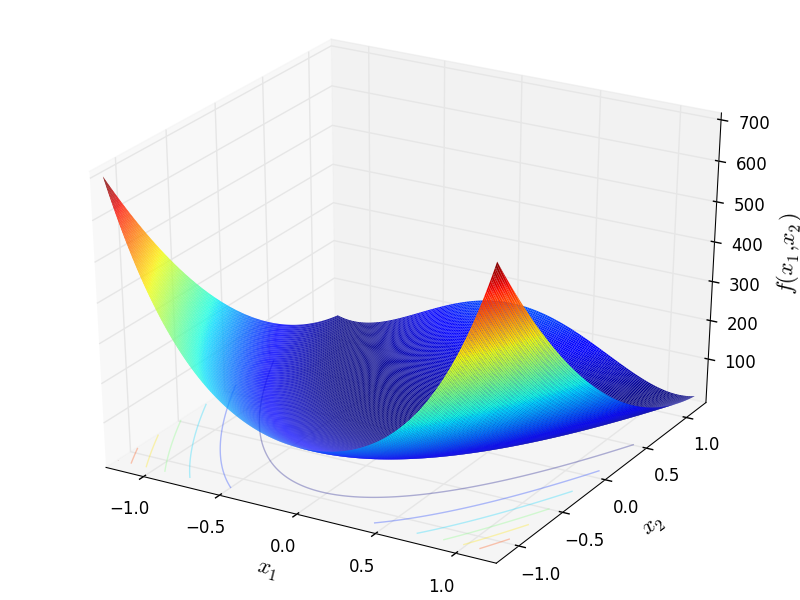Two-dimensional Leon function

Global optimum: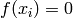for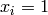forclass go_benchmark.Levy03(dimensions=2)

Levy 3 test objective function.

This class defines the Levy 3 global optimization problem. This is a multimodal minimization problem defined as follows: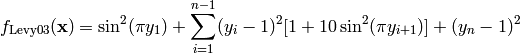Where, in this exercise: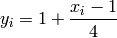Here,represents the number of dimensions andfor.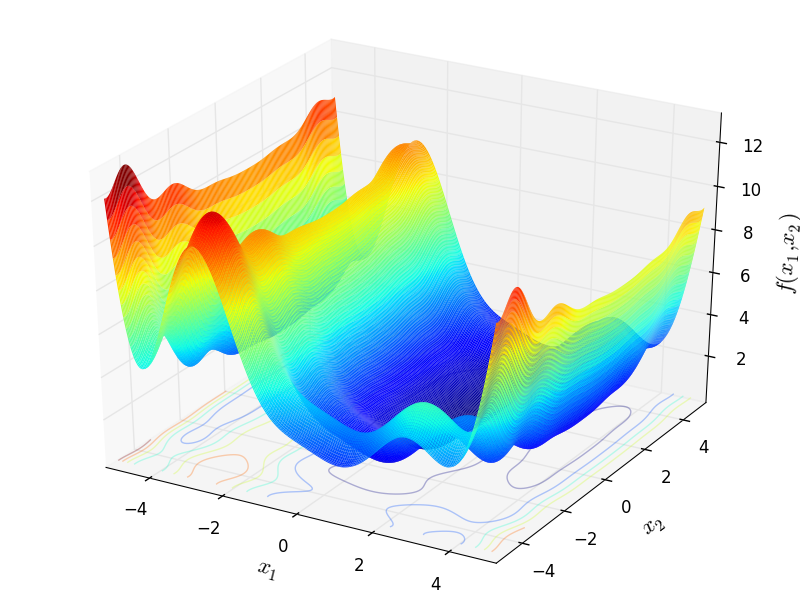Two-dimensional Levy 3 function

Global optimum:forforclass go_benchmark.Levy05(dimensions=2)

Levy 5 test objective function.

This class defines the Levy 5 global optimization problem. This is a multimodal minimization problem defined as follows: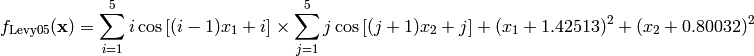Here,represents the number of dimensions andfor.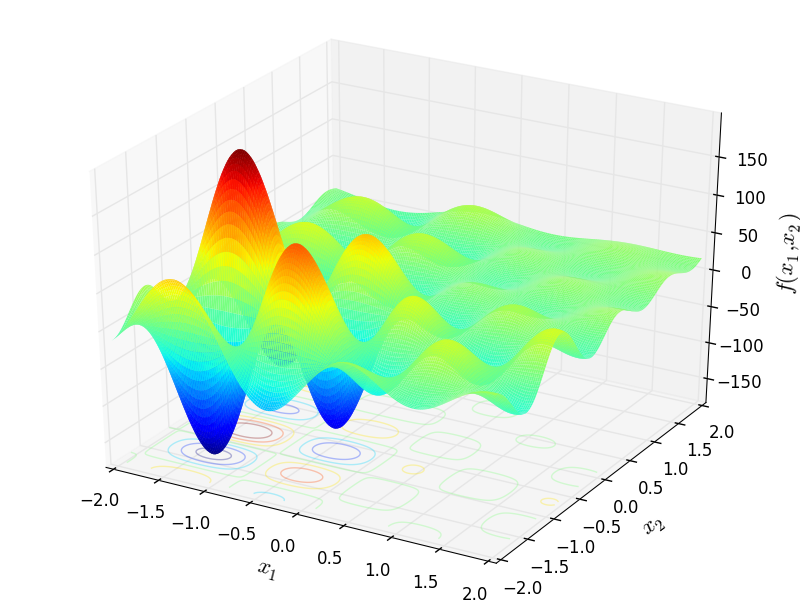Two-dimensional Levy 5 function

Global optimum:for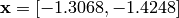.

class go_benchmark.Levy13(dimensions=2)

Levy13 test objective function.

This class defines the Levy13 global optimization problem. This is a multimodal minimization problem defined as follows: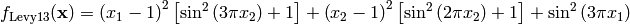Here,represents the number of dimensions andfor.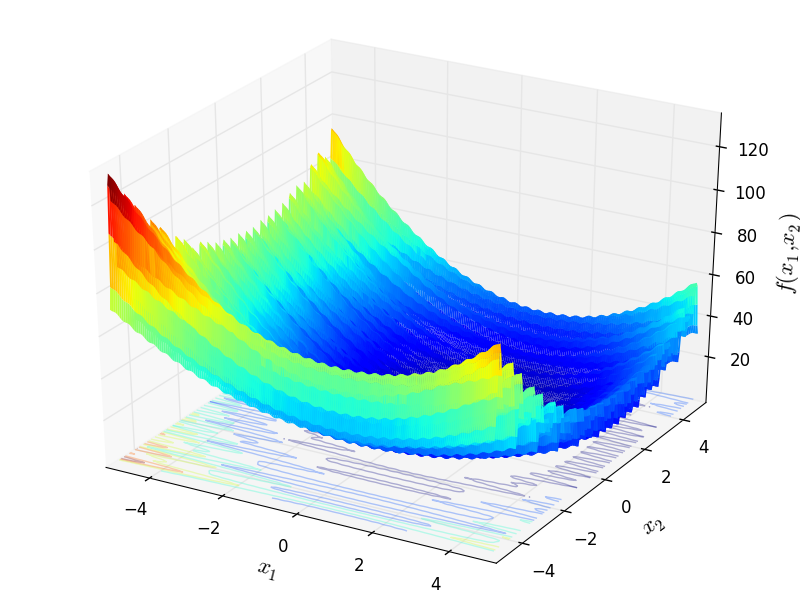Two-dimensional Levy13 function

Global optimum:forfor#### Previous topic

N-D Test Functions K

#### Next topic

N-D Test Functions M